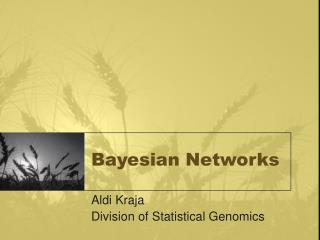DownloadDownload PresentationBayesian Networks

# Bayesian Networks

Télécharger la présentation## Bayesian Networks

- - - - - - - - - - - - - - - - - - - - - - - - - - - E N D - - - - - - - - - - - - - - - - - - - - - - - - - - -
##### Presentation Transcript

1. Bayesian Networks Aldi Kraja Division of Statistical Genomics

2. Bayesian Networks and Decision Graphs. Chapter 1 • Causal networks are a set of variables and a set of directed links between variables • Variables represent events (propositions) • A variable can have any number of states • Purpose: Causal networks can be used to follow how a change of certainty in one variable may change certainty of other variables

3. Causal networks Y, N Fuel Y, N Clean Sparks F, ½, E Y, N Start Fuel Meter Standing Causal Network for a reduced start car problem

4. Causal Networks and d-separation • Serial connection (blocking) A B C Evidence maybe transmitted through a serial connection unless the state of the variable in the connection is known. A and C and are d-separated given B When B is instantiated it blocks the communication between A and C

5. Causal networks and d-separation • Diverging connections (Blocking) A B C E … Influence can pass between all children of A unless the state of A is known Evidence may be transmitted through a diverging connection unless it is instantiated.

6. Causal networks and d-separation • Converging connections (opening) Evidence may only be transmitted through the converging connection If either A or one of its descendants has received evidence B C … E A Case1: If nothing is known about A, except inference from knowledge of its parents => then parents are independent Evidence on one of the parents has no influence on other parents Case 2: If anything is known about the consequences, then information in one may tell us something about the other causes. (Explaining away effect)

7. Evidence • Evidence on a variable is a statement of the certainties of its states • If the variable is instantiated then the variable provides hard evidence • Blocking in the case of serial and diverging connections requires hard evidence • Opening in the case of converging connections holds for all kind of evidence

8. D-separation • Two distinct variables A and B in a causal network are d-separated if, for all paths between A and B there is an intermediate variable V (distinct from A and B) such that: • -The connection is SERIAL or DIVERGING and V is instantiated • Or • - the connection is CONVERGING and neither V nor any of V’s descendants have received evidence

9. Probability Theory • The uncertainty raises from noise in the measurements and from the small sample size in the data. • Use probability theory to quantify the uncertainty. ripe Wheat unripe Wheat P(B=g)=6/10 Red fungus Gray fungus P(B=r)=4/10

10. Probability Theory • The probability of an event is the fraction of times that event occurs out of the total number of trails, in the limit that the total number of trails goes to infinity

11. Probability Theory • Sum rule: • Product rule i=1 …… M ci j=1 …… L Y=yi nij rj X=xi

12. Probability Theory i=1 …… M ci j=1 …… L Y=yi nij rj X=xi

13. Probability Theory • Symmetry property

14. Probability Theory • P(W=u | F=R)=8/32=1/4 • P(W=r | F=R)=24/32=3/4 • P(W=u | F=G)=18/24=3/4 • P(W=r | F=G)=6/24=1/4 1 1 ripe Wheat unripe Wheat P(F=G)=6/10 =0.6 Red fungus Gray fungus P(F=R)=4/10=0.4

15. Probability Theory • p(W=u)=p(W=u|F=R)p(F=R)+p(W=u|F=G)p(F=G) =1/4*4/10+3/4*6/10=11/20 • p(W=r)=1-11/20=9/20 • p(F=R|W=r)=(p(W=r|F=R)p(F=R)/p(W=r))= • 3/4*4/10*20/9=2/3 • P(F=G|W=u)=1-2/3=1/3 ripe Wheat unripped Wheat P(F=G)=6/10 =0.6 Red fungus Gray fungus P(F=R)=4/10=0.4

16. Conditional probabilities • Convergence connection (blocking) • p(a|b)p(b)=p(a,b) • p(a|b,c)p(b|c)=p(a,b|c) • p(b|a)=p(a|b)p(b)/p(a) • p(b|a,c)=p(a|b,c)p(b|c)/p(a|c) a╨c | b b b a c a c p(a,b,c)=p(a|b)p(c|b)p(b) p(a,b,c)/p(b)=p(a|b)p(c|b)p(b)/p(b)

17. Conditional probabilities • Serial connection (blocking) • p(a|b)p(b)=p(a,b) • p(a|b,c)p(b|c)=p(a,b|c) • p(b|a)=p(a|b)p(b)/p(a) • p(b|a,c)=p(a|b,c)p(b|c)/p(a|c) p(a,b,c)=p(a)p(b|a)p(c|b) p(a,c|b)=p(a,b,c)/p(b)= p(a)p(b|a)p(c|b)/p(b)= p(a) {p(a|b)p(b)/p(a)} p(c|b)/p(b)=p(a|b)p(c|b) a╨c | b a b c a b c

18. Conditional probabilities • Convergence connection (opening) • p(a|b)p(b)=p(a,b) • p(a|b,c)p(b|c)=p(a,b|c) • p(b|a)=p(a|b)p(b)/p(a) • p(b|a,c)=p(a|b,c)p(b|c)/p(a|c) a╨c | 0 p(a,b,c)=p(a)p(c)p(b|a,c) p(a,c|b)=p(a,b,c)/p(b)= p(a)p(c)p(b|a,c)/p(b) a╨c | b c a a c b b

19. Graphical Models • We need probability theory to quantify the uncertainty. All the probabilistic inference can be expressed with the sum and the product rule. p(a,b,c)=p(c|a,b)p(a,b) DAG a b p(a,b,c)=p(c|a,b)p(b|a)p(a) c P(x1,x2,….,xK-1,xK)=p(xK|x1,...,xK-1)…p(x2|x1)p(x1)

20. Graphical Models • DAG explaining joint distribution of x1,…x7 • The joint distribution defined by a graph is given by the product, over all of the nodes of a graph, of a conditional distribution of each node conditioned on the variables corresponding to the parents of that node in the graph. x1 x2 x3 x4 x5 x6 x7